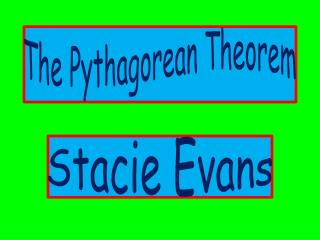DownloadDownload PresentationThe Pythagorean Theorem

# The Pythagorean Theorem

Download Presentation## The Pythagorean Theorem

- - - - - - - - - - - - - - - - - - - - - - - - - - - E N D - - - - - - - - - - - - - - - - - - - - - - - - - - -
##### Presentation Transcript

1. The Pythagorean Theorem Stacie Evans

2. What is the Pythagorean Theorem? • The Pythagorean Theorem relates the side lengths of a right triangle. • In any right triangle, the sum of the squares of the lengths of the two legs is equal to the square of the length of the hypotenuse. a² + b² = c² HYPOTENUSE a c b LEGS

3. http://web.rollins.edu/~jsiry/PythagoreanTriTheorem.jpg

4. Use the Pythagorean Theorem to solve the following problems.

5. BASEBALL MATH How far does the second baseman have to throw the ball in order to get the runner out before he slides into the home plate? (Round to the nearest whole number.)

6. In a baseball diamond, the distance between each of the three bases and home plate are 90 feet and all form right angles. Therefore, you can use the Pythagorean Theorem to solve the question. 2nd 3rd 1st BASE

7. The Equation a² + b² = c² (90)² + (90)²= c² 8100 + 8100 = c² 16,200 = c² (√16,200) = (√c²) 127 = c

8. The Final Answer The second baseman would have to throw the baseball 127 feet for the catcher to catch it before the runner slides onto home plate.

9. Television Math • Television sets are generally classified diagonally. http://www.avland.co.uk/panasonic/tx32lxd500/tx32lxd500lrg.jpg

10. The Problem • You want to purchase an entertainment center, but it holds only enough room in its cubicle for a 27 inch TV set. • The length of your TV is 15 inches, and the height of your TV is 12 inches. • The Question: • Will your TV fit into the cubicle?

11. ? 12 inches 15 inches

12. The Equation a² + b² = c² (12)² + (15)² = c² 144 + 225 = c² 369 = c² (√369) = (√c²) 19.2 = c

13. The Final Answer • The television is 19.2 inches. The television will fit into the 27 inch TV center.

14. The Miles from Where You Are • Cinderella and Prince Charming are meeting at the Palace on the corner of Perfect and Pretty Street. Cinderella is on Perfect Street and is 8 miles from the corner. Meanwhile, Prince Charming is on Pretty Street and is 7 miles from the corner. They are desperate to know how far away they are from each other. Can you find out how far apart they are?

15. 8 miles Perfect Street ? Pretty Street 7 Miles

16. The Equation a² + b² = c² (8)² + (7)² = c² 64 + 49 = c² 113 = c² (√113) = (√c²) 10.6 = c

17. The Final Answer • Cinderella and Prince Charming are 10.6 miles apart.

18. The Helicopter Flight • A helicopter flies 10 miles due north and then 24 miles due east. Then the helicopter flies in a straight line back to its starting point. What was the distance of the helicopter’s last leg back to its starting point?

19. 24 miles 10 miles ?

20. The Equation a² + b² = c² (10)² + (24)² = c² 100 + 576 = c² 676 = c² (√676) = (√c²) 26 = c

21. The Final Answer • The distance of the helicopter’s last leg was 26 miles.

22. Land Ho How far is the sailboat from the lighthouse, to the nearest kilometer? (on the next slide)

23. 130 km 50 km ?

24. The Equation a² + b² = c² (50)² + (130)² = c² 2500 + 16900 = c² 19400 = c² (√19400) = (√c²) 139 = c

25. The Final Answer • The sailboat is 139 kilometers away from the lighthouse.

26. CONGRATULATIONS! You have mastered the Pythagorean Theorem!

27. Bibliography • http://www.suite101.com/content/the-pythagorean-theorem-a21010 • http://www.disneylandpostcards.com/images/Cinderella.jpg • http://www.ltmparty.com/images/products/DU/large/5969.jpg • http://www.wdwpublicaffairs.com/Resources/images/Cinderella_Castle_ice__36556655_500w.jpg • http://neildthompson.com/wp-content/uploads/2010/08/MP900401198.jpg http://www.stregisbalharbour.com/images/no-flash-views.jpg http://www.cksinfo.com/clipart/traffic/boats/lighthouse-01.png http://www.wpclipart.com/page_frames/school/certificate_frame.png http://www.btinternet.com/~steve.sedgwick/images/Pythagguitar/pythagoras.jpg Georgia HOLT Mathematics Course 3 Pythagoras